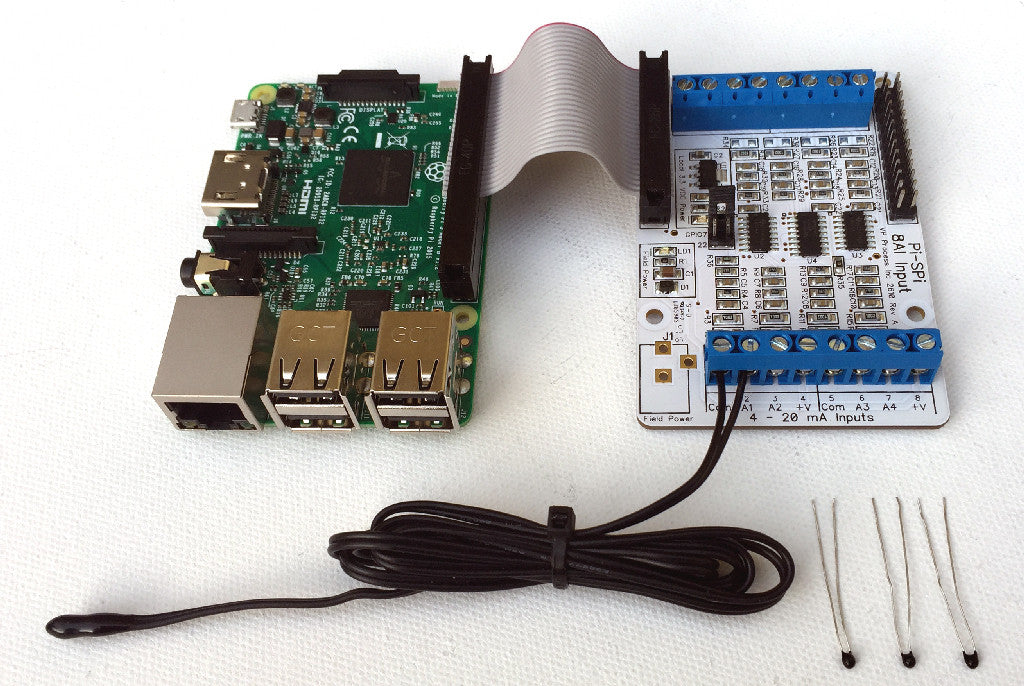# Raspberry Pi and Temperature Sensors

The most common analog input for any control and measurement system is temperature and the most common (and least expensive) temperature sensor is the thermistor. The Pi-SPi-8AI is now offered as an 8 channel thermistor temperature sensor interface.The kit includes everything required to get going with temperature measurements:

• 8 channels configured for thermistor inputs
• 40 pin to 26 pin cable to connect to the Raspberry Pi
• Qty 1 10K 3380 Beta Thermistor with 36" of lead length (Cantherm)
• Qty 3 10K 3380 Beta Thermistors (Cantherm)

The input circuits are all pre-configured and are ready to go! Each input circuit looks like this:The test code provided uses the Stein-hart Equation for simple, reliable and accurate temperature readings.

Here is part of the test code that shows how the equation works:

// Steinhar-Hart Equation Defines for 10K Thermistor Beta 3380 Temp Sensor

#define R_ROOM_TEMP        10000.0 // for 25 Deg C
#define T_BETA            3380
#define ROOM_TEMP_NOM     25.0

void Update_Temperatures(unsigned char channel) {

float sample;
unsigned int average;
int i;

// Calculate Temperature for Cold Water Line
average = 0;

for(i=0; i<5; i++) {            // take 5 reading and average
Update_Analog(channel);            // to smooth out the reading
}

average = average / 5;

sample = ((float)T_AD_COUNTS / average) - 1;
sample = sample / (float)R_ROOM_TEMP;
sample = log(sample);
sample /= (float)T_BETA;
sample += 1.0 / ((float)ROOM_TEMP_NOM +275.15);
sample = 1.0 / sample;
sample -= 273.15;

AN_Temp[channel] = sample;

}

Applications for this interface would include:

• HVAC Controls
• Hydroponics
• Refrigeration (Fridge/Freezer) Temperature Monitoring

The Pi-SPi-8AI Temperature Sensor Kit can be purchased here.

SaveSaveSave